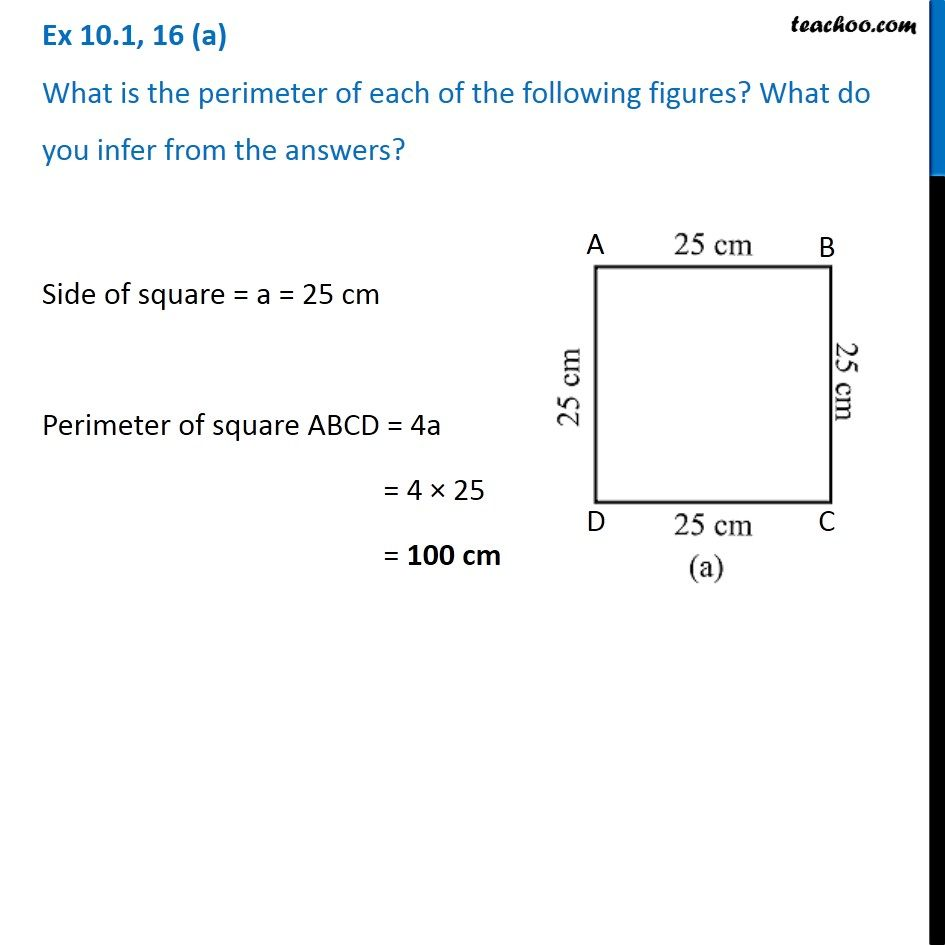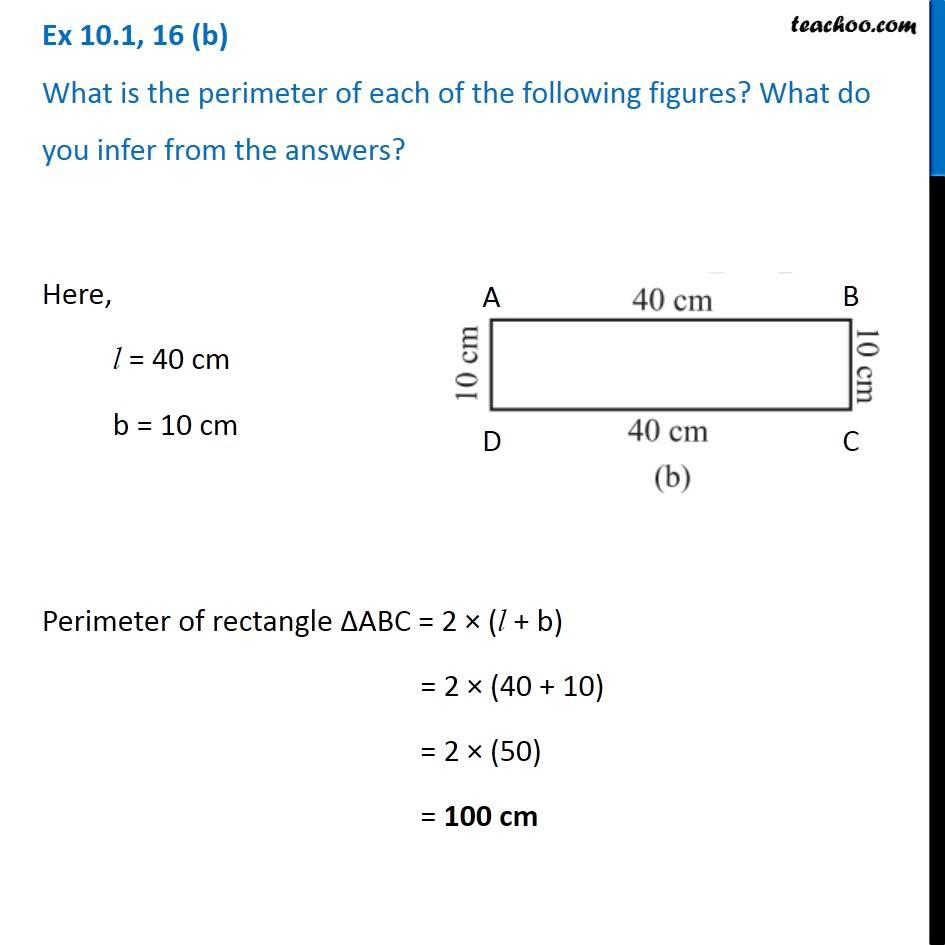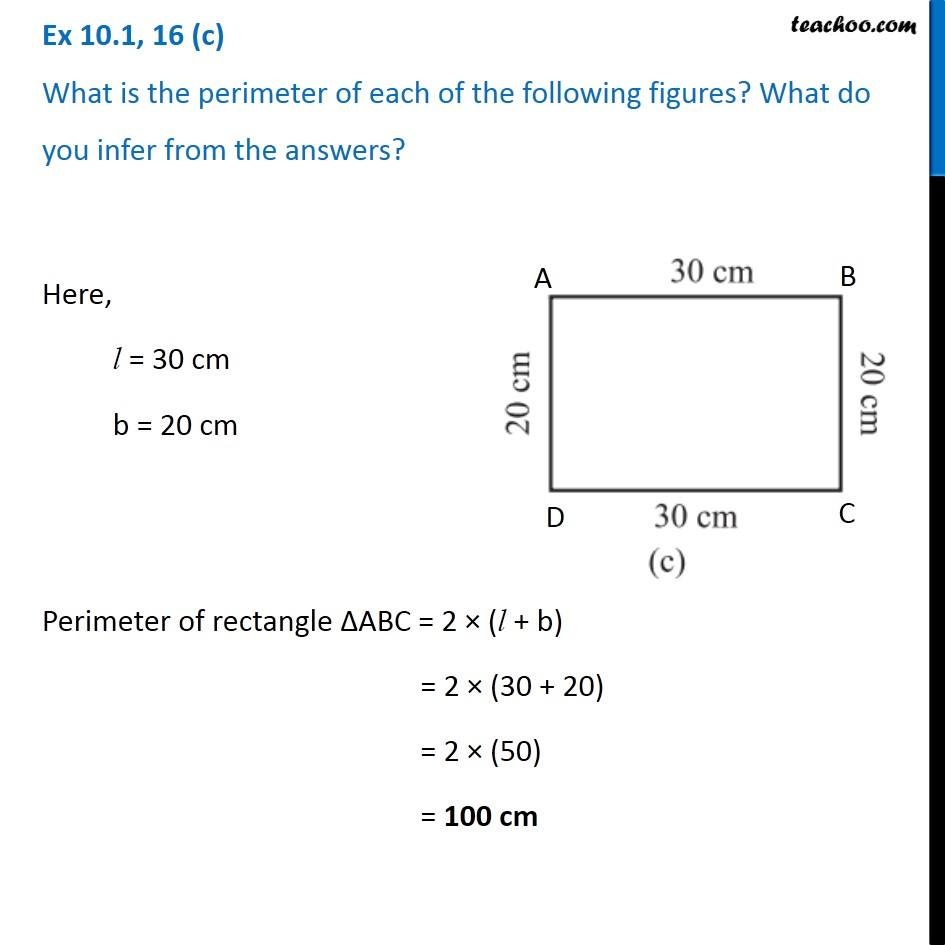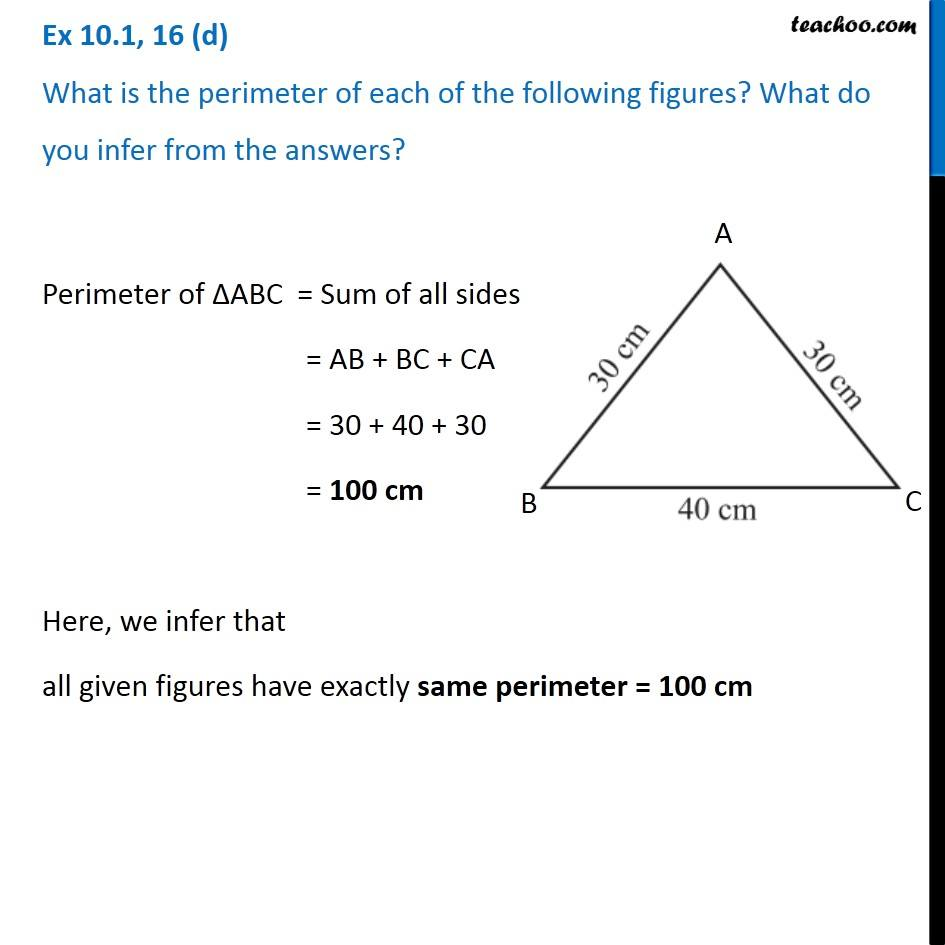Ex 10.1

Chapter 10 Class 6 Mensuration
Serial order wiseGet live Maths 1-on-1 Classs - Class 6 to 12

### Transcript

Ex 10.1, 16 (a) What is the perimeter of each of the following figures? What do you infer from the answers? Side of square = a = 25 cm Perimeter of square ABCD = 4a = 4 × 25 = 100 cm Ex 10.1, 16 (b) What is the perimeter of each of the following figures? What do you infer from the answers? Here, l = 40 cm b = 10 cm Perimeter of rectangle ∆ABC = 2 × (l + b) = 2 × (40 + 10) = 2 × (50) = 100 cm Ex 10.1, 16 (c) What is the perimeter of each of the following figures? What do you infer from the answers?Here, l = 30 cm b = 20 cm Perimeter of rectangle ∆ABC = 2 × (l + b) = 2 × (30 + 20) = 2 × (50) = 100 cm Ex 10.1, 16 (d) What is the perimeter of each of the following figures? What do you infer from the answers?Perimeter of ∆ABC = Sum of all sides = AB + BC + CA = 30 + 40 + 30 = 100 cm Here, we infer that all given figures have exactly same perimeter = 100 cm